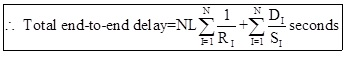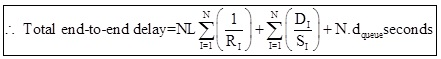SHARE
HELP

To start with, Sr2Jr’s first step is to reduce the expenses related to education. To achieve this goal Sr2Jr organized the textbook’s question and answers. Sr2Jr is community based and need your support to fill the question and answers. The question and answers posted will be available free of cost to all.Authors:
James F. Kurose, Keith W. Ross
Chapter:
Computer Networks And The Internet
Exercise:
Problems
Question:17 | ISBN:9780132856201 | Edition: 6

###Question

a. Generalize Equation 1.2 in Section 1.4.3 for heterogeneous processing rates, transmission rates,

and propagation delays.

b. Repeat (a), but now also suppose that there is an average queuing delay of dqueue at each node.

###Answer

a)

Consider Data,

Generalize Equation 1.2 in Section 1.4.3 for heterogeneous processing rates, transmission rates,and propagation delays.

Total end-to-end delay = Transmission delay + Propagation delayb)

Consider data,

Suppose that there is an average queuing delay of dqueue at each node.

Total end-to-end delay = Transmission delay + Propagation delay + Queuing delay### Discussions

Post the discussion to improve the above solution.